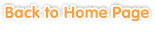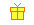Sample questions are shown below. Just click to start!• Chapter 1
Addition and Subtraction with 2-Digit Numbers
•
Number Lines•••
Subtraction without Regrouping•
Subtraction with Regrouping••
Estimating Sums and Differences• Chapter 2• Chapter 3
Multiplication Facts
•
The Relationship between Addition and Multiplication•
Multiplication Facts to 4•
Multiplication Facts to 5••
Multiplication Facts to 7•
Multiplication Facts to 8•
Multiplication Facts to 9• Chapter 4
Division Facts
•
Dividing Evenly into Groups•
The Relationship between Multiplication and Division•
Division Facts to 6•
Division Facts to 7••
Division Facts to 9• Chapter 5
Problem Soving: Multiplication and Division Facts• Chapter 6
Numbers Up to 99,999
•
Writing Numbers••
Comparing Numbers and Sequencing•
Rounding•
Adding and Subtracting 1,000s, 100s and 10s•
Estimating Sums and Differences• Chapter 7
Customary Measurement
••
Length Conversion (inches, feet, and yards)•
Weight Conversion (ounces and pounds)•• Chapter 8
Metric Measurement
•
Measuring Lengths in Centimeters•
Length Conversion (centimeters and meters)•
Weight Conversion (grams and kilograms)•• Chapter 9
Geometry
•
Lines, Rays, and Line Segments•• Chapter 10
Patterns
•
Numbers•
Colors, Shapes, and Pictures• Chapter 11
Statistics
•
Data Analysis